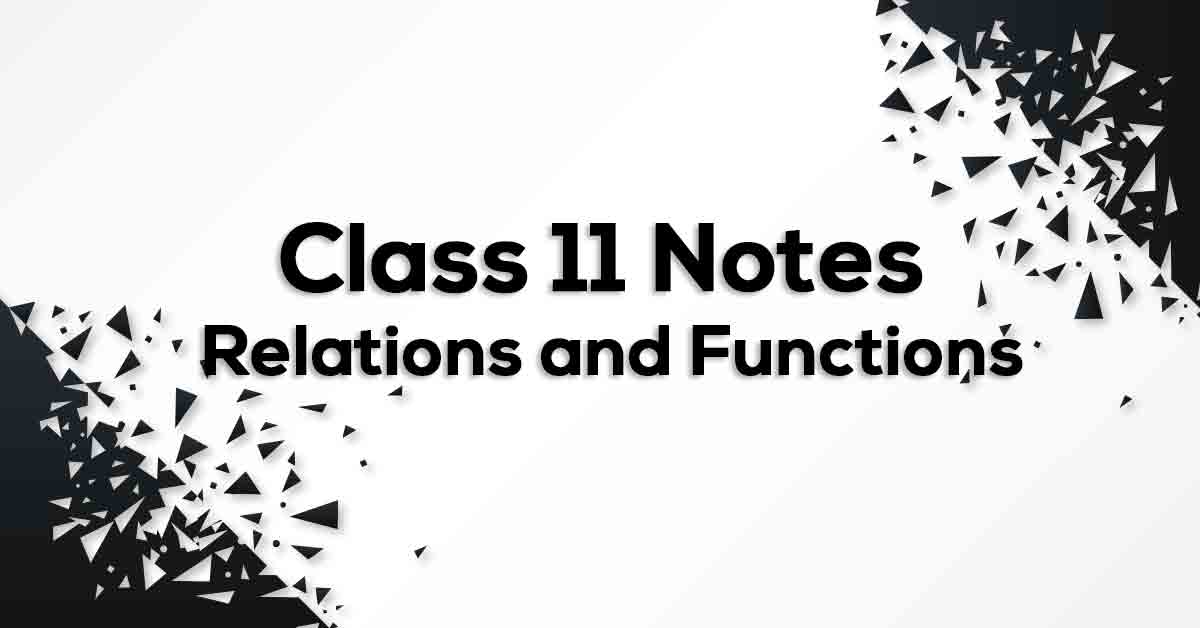Relations and Functions Class 11 Formulae | Vidyakul

Relations and Functions Class 11 FormulaeClass 11 Math Chapter 2 Relations and Functions Formulae - PDF Download

Relations and Functions Class 11 Formulae List is prepared strictly according to the NCERT Syllabus which not only reduces the pressure on the students but also, offer them a simple way to study or revise the chapter. These formulae are cumulated from past 15 years of examination material preferred by CBSE so that no important formulae should be left behind for the students to know and practice. Relations and Functions Class 11 Formulae would fuel your exam preparation and increase your self-confidence which would help you score maximum marks in the exam.

Define Relations and Functions?

A relation f from a set A to a set B is said to be function if every element of set A has one and only one image in set B. In other words, a function f is a relation such that no two pairs in the relation has the same first element. A function is a relation in which each input has only one output. In the relation, y is a function of x, because for each input x (1, 2, 3, or 0), there is only one output y. x is not a function of y, because the input y = 3 has multiple outputs: x = 1 and x = 2.